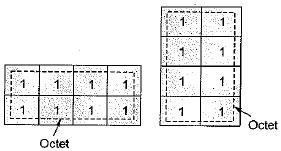Courses

# Test: Boolean Algebra & Minimization Techniques

## 10 Questions MCQ Test Topicwise Question Bank for Electrical Engineering | Test: Boolean Algebra & Minimization Techniques

Description
This mock test of Test: Boolean Algebra & Minimization Techniques for GATE helps you for every GATE entrance exam. This contains 10 Multiple Choice Questions for GATE Test: Boolean Algebra & Minimization Techniques (mcq) to study with solutions a complete question bank. The solved questions answers in this Test: Boolean Algebra & Minimization Techniques quiz give you a good mix of easy questions and tough questions. GATE students definitely take this Test: Boolean Algebra & Minimization Techniques exercise for a better result in the exam. You can find other Test: Boolean Algebra & Minimization Techniques extra questions, long questions & short questions for GATE on EduRev as well by searching above.
QUESTION: 1

### Three Boolean operators are

Solution:

The three Boolean operators are NOT, OR, AND, These are also called basic gates for digital electronics.

QUESTION: 2

### The only function of a NOT gate is to

Solution: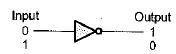NOT gate is used to invert the input signal.

QUESTION: 3

### Negative logic in 'a logic circuit is one in which

Solution:

In negative logic:

• logic 0 = Some positive voltage
• logic 1 = Zero voltage

or

• logic 0 = Some higher voltage level (i.e. + 5 V,)
• logic 1 = Some lower voltage level (i.e. + 2 V)
QUESTION: 4

Which of the following Boolean Expressions is not true?

Solution:

A + 1 = 1 (Always)
Hence,  option (c) is not correct.

QUESTION: 5

The simplified form of the Boolean expression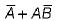is

Solution:

Using distribution theorem, we have: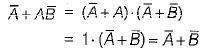QUESTION: 6

The Boolean expression: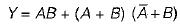may be simplified as

Solution:

Given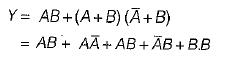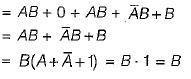QUESTION: 7

The complement form of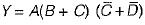is

Solution:

Given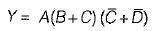∴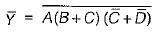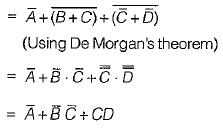QUESTION: 8

The POS form of expression is suitable for circuit using:

Solution:

POS form (Product of Sum form) of expression is suitable for circuit using NOR gates.

QUESTION: 9

Which of the following is/are the correct reason for using Gray code in K-maps?

Solution:
QUESTION: 10

When eight adjacent 1 's are grouped horizontally or vertically the group so found is called

Solution: